Latest Banking jobs   »   Quantitative Aptitude Quiz For ESIC- UDC,...

# Quantitative Aptitude Quiz For ESIC- UDC, Steno, MTS Prelims 2022-30th December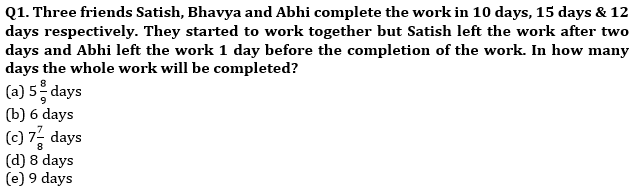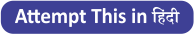Q2. ⅔rd of first number is equal to the cube of the second number. If the second number is equal to 12% of 100, what is sum of the first & 2nd number?
(a) 2408
(b) 2640
(c) 2426
(d) 2604
(e) 2804

Q3. A wholeseller sells an item to a retailer at 20% discount, but charges 10% on the discounted price for packaging & delivery. The retailer sells it for 1023 more, thereby earning a profit of 25%. At what price had the wholeseller marked the item ?
(a) Rs. 4620
(b) Rs. 4650
(c) Rs. 4850
(d) Rs. 5240
(e) Rs. 5445

Q4. The present age of Bhagat and Abhi are in ratio of 9 : 8 respectively. After 10 years the ratio of their ages will be 10 : 9. What is the difference in their present age ?
(a) 8 years
(b) 6 years
(c) 12 years
(d) 4 years
(e) 10 years

Q5. The circumference of two circles is 132 m and 176 m respectively. What is difference between the area of larger circle and smaller circle ? (in m²)
(a) 1052
(b) 1128
(c) 1258
(d) 1078
(e) 1528

Q6. Diameter of a cylindrical jar is increased by 25%. By what percent must the height be decreased so that there is no change in its volume?
(a) 18%
(b) 25%
(c) 32%
(d) 36%
(e) None of these

Q7. If income & saving ratio of a person is 25 : 9. If saving increases ⅓rd & expenditure increases by 25% & income increases by Rs. 2100. Then find the initial saving?
(a) Rs. 3200
(b) Rs. 3500
(c) Rs. 5600
(d) Rs. 2700
(e) Rs. 4200

Q8. Ayush can do a work in 40 days and Rahul is 25% more efficient than Ayush. If Ayush started and worked for 15 days and left then in how many days will Rahul do the remaining work ?
(a) 16 days
(b) 20 days
(c) 28 days
(d) 24 days
(e) 12 days

Q9. If speed of boat in upstream is double than the speed of current and speed of boat in still water is 27 km/hr. Then find the time taken by boat to travel 54 km downstream? (in hour)
(a) 1.5
(b) 1.8
(c) 2.5
(d) 1.2
(e) 2

Q10. Sum of four consecutive even numbers is 49 more than sum of three consecutive odd numbers and the sum of lowest odd and lowest even number is 23. Find the largest even number.
(a) 28
(b) 36
(c) 18
(d) 32
(e) 22

Q11. A leak in the bottom of a tank can empty the full tank in 20 hrs. If both inlet pipe and leak is opened then the tank filled in 60 hrs. Find the capacity of tank if inlet pipe can fill at the rate of 4 ℓ per minute.
(a) 1800 ℓ
(b) 2400 ℓ
(c) 2700 ℓ
(d) 3600 ℓ
(e) 4500 ℓ

Q12. Average age of a class of 15 student is 18 years. After admission of 5 new student average age of class decreases by 0.25. Find the average age of the 5 new students.
(a) 16.5
(b) 17
(c) 17.25
(d) 17.5
(e) 17.75

Q13. A train covers certain distance between two places at a uniform speed. If the train moved 10 kmph faster, it would take 2 hours less, and if the train were slower by 10 kmph, it would take 3 hours more than the scheduled time. Find the distance covered by the train.
(a) 300 km
(b) 600 km
(c) 800 km
(d) 1200 km
(e) None of these

Q14. Two letters are chosen out of the alphabets of the English language. Find the probability that both the letters are vowels.
(a) 2/65
(b) 3/65
(c) 1/65
(d) 3/5
(e) 7/65

Q15. How many five-letters containing 2 vowels and 3 consonants can be formed using the letters of the word EQUALITY so that 2 vowels occur together?
(a) 1260
(b) 1000
(c) 1150
(d) 1152
(e) None of these

Solutions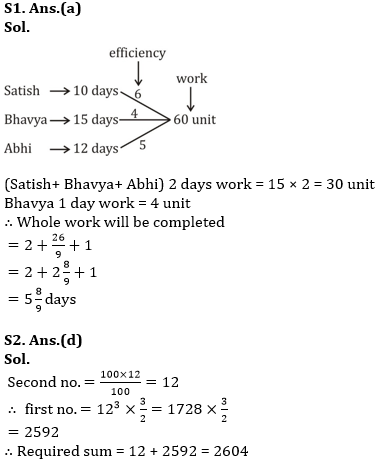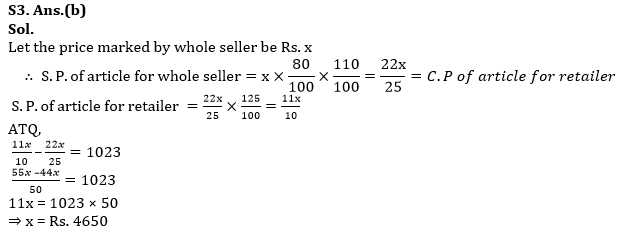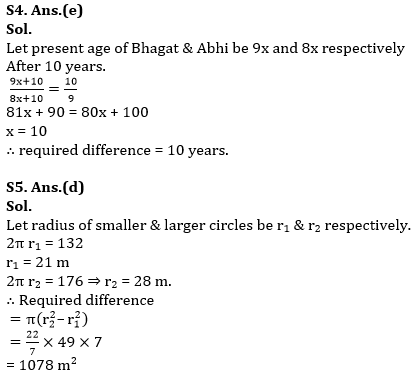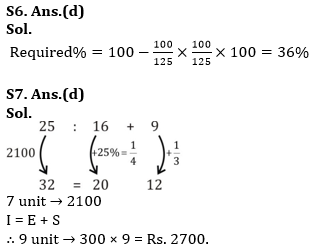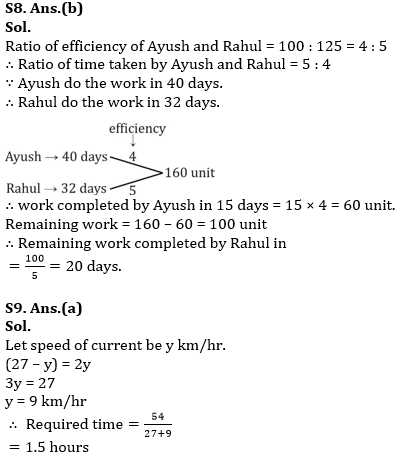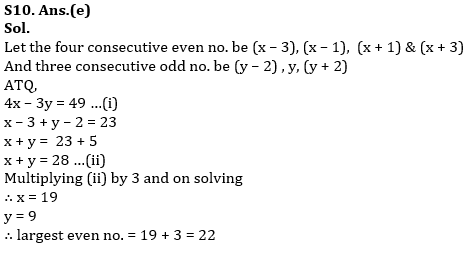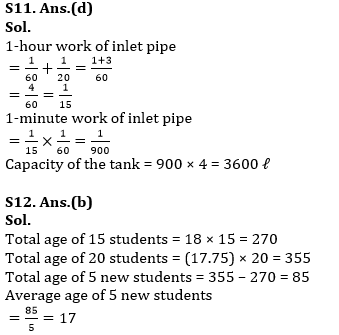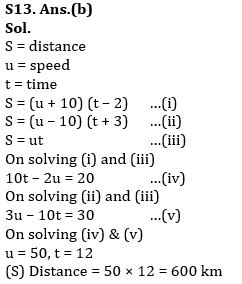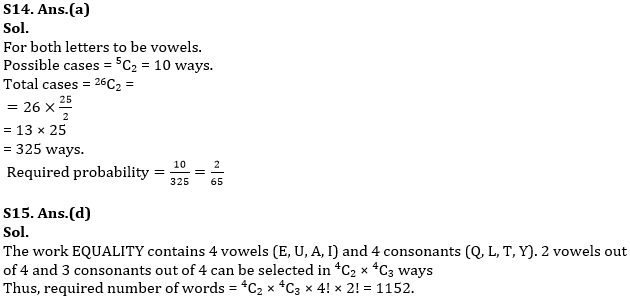#### Congratulations!Incorrect details? Fill the form again here

•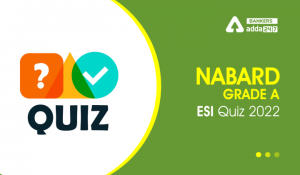ESI Quizzes For NABARD Grade A 2022- 18t...
•ESI Quizzes For NABARD Grade A 2022- 15t...
•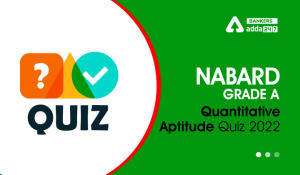Quantitative Aptitude Quiz For NABARD Gr...
•ESI Quizzes For NABARD Grade A 2022- 8th...
•ESI Quizzes For NABARD Grade A 2022- 4th...
•Quantitative Aptitude Quiz For NABARD Gr...